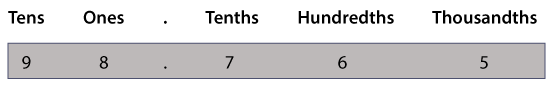Decimal place value

The number 98.765 can be placed on a place value chart like this:Example

Let's look at the number 26.5

Here is the number twenty-six and 5 tenths written as a decimal number: 26.5

The decimal point is between the ones and tenths.

26.5 has 2 tens, 6 ones and 5 tenths.

26.5 = 20 + 6 + 5/10